﻿ k-Generalized Fibonacci Numbers

### k-Generalized Fibonacci Numbers

Yashwant K. Panwar, Mamta SinghOPEN ACCESSPEER-REVIEWED

## k-Generalized Fibonacci Numbers

Yashwant K. Panwar1,, Mamta Singh2

1Department of Mathematics and MCA, Mandsaur Institute of Technology, Mandsaur, India

2Department of Mathematical Sciences and Computer Application, Bundelkhand University, Jhansi (U. P.), India

### Abstract

In this paper, we present the k-Generalized Fibonacci sequence. This sequence generalizes other, Generalized Fibonacci sequence. Generalized Fibonacci sequence was introduced by Gupta, Panwar and Sikhwal in 2012. We establish some of the interesting properties of k- Generalized Fibonacci sequence.

### Cite this article:

• Panwar, Yashwant K., and Mamta Singh. "k-Generalized Fibonacci Numbers." Applied Mathematics and Physics 2.1 (2014): 10-12.
• Panwar, Y. K. , & Singh, M. (2014). k-Generalized Fibonacci Numbers. Applied Mathematics and Physics, 2(1), 10-12.
• Panwar, Yashwant K., and Mamta Singh. "k-Generalized Fibonacci Numbers." Applied Mathematics and Physics 2, no. 1 (2014): 10-12.

 Import into BibTeX Import into EndNote Import into RefMan Import into RefWorks

### 1. Introduction

It is well-known that the Fibonacci sequence is most prominent examples of recursive sequence. The Fibonacci sequence is famous for possessing wonderful and amazing properties. The Fibonacci appear in numerous mathematical problems. Fibonacci composed a number text in which he did important work in number theory and the solution of algebraic equations. Many authors have generalized second order recurrence sequences by preserving the recurrence relation and alternating the first two terms of the sequence and some authors have generalized these sequences by preserving the first two terms of the sequence but altering the recurrence relation slightly.

The k-Fibonacci numbers defined by Falco’n and Plaza , depending only on one integer parameter k as follows,

For any positive real number k, the k-Fibonacci sequence is defined recurrently by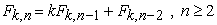(1.1)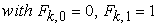.

Many of the properties of these sequences are proved by simple matrix algebra. This study has been motivated by the arising of two complex valued maps to represent the two antecedents in an specific four-triangle partition. In , Falcon and Plaza k- Fibonacci sequence generalizes, between others, both the classical Fibonacci sequence and the Pell sequence. In this paper many properties of these numbers are deduced and related with the so-called Pascal 2-triangle. New generalized k-Fibonacci sequences have been introduced and studied. Several properties of these numbers are deduced and related with the so-called Pascal 2-triangle. In addition, the generating functions for these k-Fibonacci sequences have been given.

The Generalized Fibonacci sequences , defined by Gupta, Panwar and Sikhwal, as follows,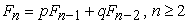(1.2)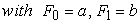.

If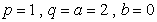, we get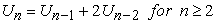(1.3)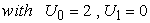.

The first few terms of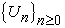are 2, 0, 4, 4, 12, 20 and so on.

In this paper, we introduced the k-Generalized Fibonacci sequence. Also we establish some of the interesting properties of k-Generalized Fibonacci numbers. In this paper, we present properties of k-Generalized Fibonacci numbers like Catalan’s identity, Cassini’s identity and d’ocagnes’s Identity.

### 2. The k-Generalized Fibonacci Numbers

In this section, we define k-generalized Fibonacci sequence. For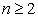, the k-generalized Fibonacci sequence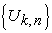is defined by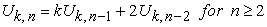(2.1)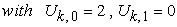.

The first few k-generalized Fibonacci numbers are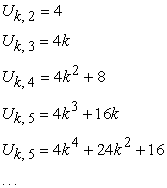Particular case of k-generalized Fibonacci number is

If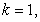generalized Fibonacci sequence is obtained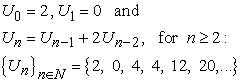### 3. Properties of k-Generalized Fibonacci Numbers

3.1. First Explicit Formula for k-Generalized Fibonacci Numbers

In the 19th century, the French mathematician Binet devised two remarkable analytical formulas for the Fibonacci and Lucas numbers. In our case, Binet’s formula allows us to express the generalized k-Fibonacci numbers in function of the roots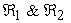of the following characteristic equation, associated to the recurrence relation (2.1)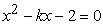(3.1)

Theorem 1: (Binet’s formula). The nth k-generalized Fibonacci number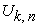is given by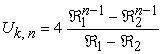(3.2)

whereare the roots of the characteristic equation (3.1) and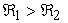.

Proof: We use the Principle of Mathematical Induction (PMI) on n. It is clear the result is true for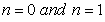by hypothesis. Assume that it is true for r such that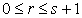, then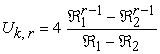It follows from definition of k-generalized Fibonacci numbers (2.1) and equation (3.2)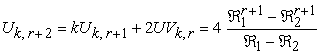Thus, the formula is true for any positive integer n.

where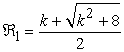and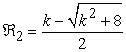.

This completes the proof.

3.2. Catalan's Identity

Catalan's identity for Fibonacci numbers was found in 1879 by Eugene Charles Catalan a Belgian mathematician who worked for the Belgian Academy of Science in the field of number theory.

Theorem 2: (Catalan’s identity)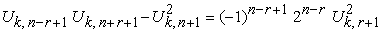(3.3)

Proof: By Binet’s formula (3.2), we have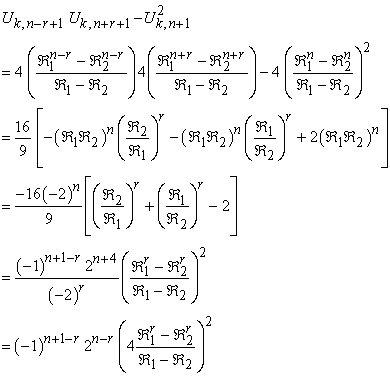This completes the Proof.

3.3. Cassini's Identity

This is one of the oldest identities involving the Fibonacci numbers. It was discovered in 1680 by Jean-Dominique Cassini a French astronomer.

Theorem 3: (Cassini’s identity or Simpson’s identity)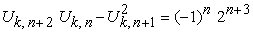(3.4)

Proof. Taking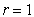in Catalan’s identity (3.3) the proof is completed.

3.4. D'Ocagne's Identity

Theorem 4: (d’ocagnes’s Identity)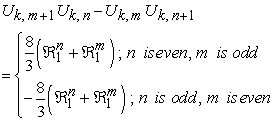(3.5)

Proof. By Binet’s formula (3.2), we have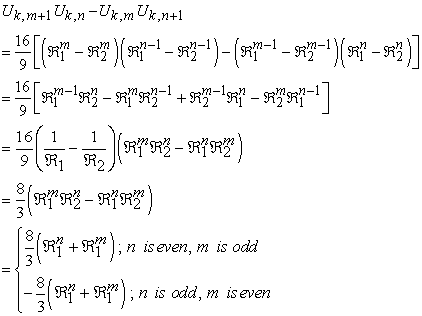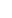This completes the Proof.

3.5. Limit of the Quotient of Two Consecutive Terms

A useful property in these sequences is that the limit of the quotient of two consecutive terms is equal to the positive root of the corresponding characteristic equation

Theorem 5: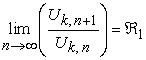(3.6)

Proof. By Binet’s formula (3.2), we have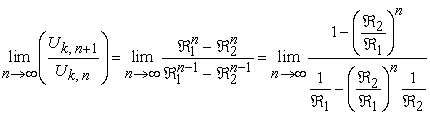and taking into account that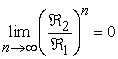, since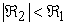, Eq. (3.6) is obtained.

3.6. Generating function for the k-Generalized Fibonacci Sequence:

Generating functions provide a powerful technique for solving linear homogeneous recurrence relations. Even though generating functions are typically used in conjunction with linear recurrence relations with constant coefficients, we will systematically make use of them for linear recurrence relations with non constant coefficients. In this paragraph, the generating function for k-generalized Fibonacci sequence is given. As a result, k-generalized Fibonacci sequence is seen as the coefficients of the corresponding generating function. Function defined in such a way is called the generating function of the k-generalized Fibonacci sequence. So,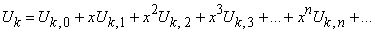and then,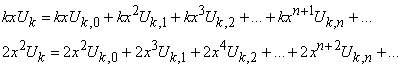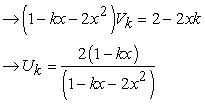(3.7)

### 4. Conclusion

In this paper new k-generalized Fibonacci sequence have been introduced and studied. Many of the properties of this numbers are proved by simple algebra and Binet’s formula. Finally we present properties like Catalan’s identity, Cassini’s identity or Simpson’s identity and d’ocagnes’s identity for k-generalized Fibonacci numbers.

### References

  A. F. Horadam, A generalized Fibonacci sequence. Math Mag., 68 (1961), 455-459.In article  Hoggatt, V.E., Fibonacci and Lucas numbers. Palo Alto, CA: Houghton, (1969).In article  Hoggatt, V.E. Jr., Phillips, J.W. and Leonard, H. Jr., “Twenty-four Master Identities”, The Fibonacci Quarterly, Vol.9, No.1, (1971), 1-17.In article  S. Falco´n, On the k-Lucas numbers. International Journal of Contemporary Mathematical Sciences, 6 (21) (2011), 1039-1050.In article  S. Falco´n, On the Lucas Triangle and its Relationship with the k-Lucas numbers. Journal of Mathematical and Computational Science, 2 (3) (2012), 425-434.In article  S. Falco´n, Plaza, A.: On the Fibonacci k-numbers. Chaos, Solitons & Fractals, 32 (5) (2007), 1615-1624.In article CrossRef  S. Falco´n, Plaza, A.: The k-Fibonacci hyperbolic functions. Chaos, Solitons & Fractals, 38 (2) (2008), 409-20.In article CrossRef  S. Falco´n, Plaza, A.: The k-Fibonacci sequence and the Pascal 2-triangle. Chaos, Solitons & Fractals, 33 (1) (2007), 38-49.In article CrossRef  S. Vajda, Fibonacci and Lucas numbers, and the golden section. Theory and applications. Chichester: Ellis Horwood limited (1989).In article  T. Koshy, Fibonacci and Lucas Numbers with Applications, John Wiley, New York. (2001).In article CrossRef  V. K. Gupta and Y. K. Panwar “Common factors of generalized Fibonacci, Jacobstha and Jacobsthal-Lucas numbers”, International Journal of Applied Mathematica Research, Vol.1, No.4 (2012), 377-382.In article  V. K. Gupta, Y. K. Panwar and O. Sikhwal, “Generalized Fibonacci sequences” Theoretical Mathematics & Applications, Vol.2, No.2 (2012), 115-124.In article